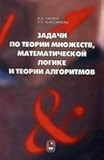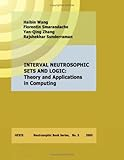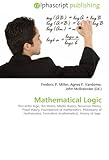Home  - Pure_And_Applied_Math - Logic And Set Theory
e99.com Bookstore
 Images Newsgroups
 Page 4     61-73 of 73    Back | 1  | 2  | 3  | 4

Logic And Set Theory:     more books (100)
1. Problems in the theory of sets, Math. logic and theory. ALG-mov / Zadachi po teorii mnozhestv, mat. logike i teor. alg-mov by Maksimova L.L. Lavrov I.A., 2009
2. Set Theory and Its Logic. Revised Edition by Willard Van Orman Quine, 1971
3. Logic and Set Theory by Philip M. And Frank J. Avenoso Cheifetz, 1970
4. Interval Neutrosophic Sets and Logic: Theory and Applications in Computing by Haibin Wang; Florentin Smarandache; Yan-Qing Zhang; Rajshekhar Sunderraman, 2005-05-31
5. Logic and Set Theory with Applications by Avenoso, Delitsky Cheifetz, 2009
6. Mathematical Logic: First-order logic, Set theory, Model theory, Recursion theory, Proof theory, Foundations of mathematics, Philosophy of mathematics, Formalism (mathematics), History of logic
7. Logic and set theory: With an introduction to computer programming by Philip M Cheifetz, 1983
8. Logic and set theory by Maurice D Weir, 1972
9. Elements of Mathematical Logic and Set Theory by Slupecki J. L. Borkowski O. Wojtasiewicz Trans.,
10. Computational Logic and Set Theory (Texts in Computer Science) by Jacob Schwartz, Eugenio Omodeo, et all 2005-05
11. Elements of Mathematical Logic and Set Theory by J.; Borkowski, L. Slupecki, 1967
12. Elements of mathematical logic and set theory by Jerzy Sl/upecki, 1967
13. Logic and set theory: A programmed approach by Philip M Cheifetz, 1969
14. Topology, logic and set theory I (Internal report) by Gary G Miller, 198861. LECTURES IN LOGIC & SET THEORY (Adobe Reader) Ebook Tourlakis, George Diesel EBo
http://www.diesel-ebooks.com/cgi-bin/item/0511060270/Lectures-in-Logic-and-Set-T

62. Algebraic Set Theory
Algebraic set theory and the effective topos. Journal of Symbolic logic, 70(3)879890, 2005. Alex Simpson. Constructive set theories and their
http://www.phil.cmu.edu/projects/ast/
Algebraic Set Theory
Algebraic set theory uses the methods of category theory to study elementary set theory. The purpose of this website is to link together current research in algebraic set theory and make it easily available. It is hoped that this will encourage and facilitate further development of the subject. Why do you call it "algebraic set theory"?
Bibliography
The following is a brief survey of the current literature on algebraic set theory. The bibliography is ordered chronologically by year of publication and then alphabetically by the author's surname (by the first author's surname in the case of works with multiple authors). Draft and preprint versions of papers are listed as they become available. Upon publication preprints are removed and papers are listed under year of publication. In most cases preprints are still available on individual author homepages or on the arXiv.

63. LPSG Set Theory And Further Logic
This paper covers basic axiomatic set theory plus a subject within the ambit of formal logic but beyond the introductory logic of a standard first year
http://www.ucl.ac.uk/philosophy/LPSG/SetTheory.htm
SET THEORY AND FURTHER LOGIC
Last Updated 28/04/05 BACK TO LPSG CONTENTS
• The Paper Textbooks
1. The Paper
This paper covers basic axiomatic set theory plus a subject within the ambit of formal logic but beyond the introductory logic of a standard first year course. In recent years that further subject has been modal logic (the logic of necessity and possibility). Another candidate for the further logic' component is intuitionistic logic, another would be probability theory. Students would of course be advised of any change in the further logic' component , and the paper in any changeover year would include questions on set theory and on both further logic' subjects. This paper has a sister paper, Mathematical Logic . The common parent was a paper, now defunct at the B.A. level, called Symbolic Logic. Once modal logic became part of the staple diet it was felt that there was too much ground for an undergraduate to cover in any depth in one paper; hence the split. The courses are taught in alternate years: if Mathematical Logic is taught one year, Set Theory and Further Logic is taught the next. But B.A. exam papers for both are set every year. What can set theory do for a philosophy student? Here is a four-part answer. (1) Set theory provides a bagful of conceptual tools useful in many areas of rigorous thought and teaches one to handle them with precision, e.g. partial ordering, equivalence relation, isomorphism, recursive definition and proof by (mathematical) induction. (2) It sharpens reflection on matters central to philosophy of thought and language, such as the nature of truth, satisfaction, predicate extensions and associated antinomies such as Russell's Paradox. (3) It is very important for philosophy of mathematics, for questions of ontology and the number systems. (4) It provides a proper understanding of infinity (and one of the deepest problems of mathematics, the continuum problem), and enables one to see through mystical twaddle wrapped in talk of the infinite.
• 64. Sets, Logic And Categories
Venn diagrams by Frank Ruskey; Beginnings of set theory (on MacTutor History of Mathematics site at St Andrews); British logic Colloquium (with many further
http://www.maths.qmul.ac.uk/~pjc/slc/
Sets, Logic and Categories
This book is published by Springer-Verlag , in the Springer Undergraduate Mathematics Series , in February 1999. Another book in the series is Geoff Smith's Introductory Mathematics: Algebra and Analysis A PDF file of the preface and table of contents is available. Solutions to the exercises (PDF files): Others to be added! Here is a list of known misprints, together with comments and improvements from various readers. From the review by A. M. Coyne in The text is clearly written. It would make an excellent first course in foundational issues in mathematics at the undergraduate level.
Further references
• Sheaves in Geometry and Logic: A first introduction to topos theory , Springer 1990. (Suggested by Steve Awodey
• A computer scientist's view: Paul Taylor, Practical Foundations of Mathematics , Cambridge University Press, 1999.
• A book about how our brains are wired to do mathematics: Brian Butterworth, The Mathematical Brain , Macmillan, London, 1999.

65. Axiomatic Set Theory - Wikipedia, The Free Encyclopedia
In mathematics, axiomatic set theory is a rigorous reformulation of set theory in firstorder logic created to address paradoxes in naive set theory.
http://en.wikipedia.org/wiki/Axiomatic_set_theory
Axiomatic set theory
Jump to: navigation search In mathematics axiomatic set theory is a rigorous reformulation of set theory in first-order logic created to address paradoxes in naive set theory . The basis of set theory was created principally by the German mathematician Georg Cantor at the end of the 19th century. Initially controversial, set theory has come to play the role of a foundational theory in modern mathematics, in the sense that it interprets propositions about mathematical objects (numbers, functions, etc.,) from all the traditional areas of mathematics ( algebra analysis topology , etc.) in a single theory, and provides a standard set of axioms to prove or disprove them. At the same time the basic concepts of set theory are used throughout mathematics, the subject is pursued in its own right as a specialty by a comparatively small group of mathematicians and logicians . It should be mentioned that there are also mathematicians using and promoting different approaches to the foundations of mathematics. The basic concepts of set theory are set and membership. A

66. IngentaConnect Should The Logic Of Set Theory Be Intuitionistic?
Many influential writers have felt that the openendedness of the set concept mandates the use of intuitionistic logic in set theory.1But with the exception
http://www.ingentaconnect.com/content/bpl/paso/2001/00000101/00000003/art00008

67. Blackwell Synergy - Proc Aristotelian Soc, Volume 101 Issue 3 Page 369-378, 2001
Your browser may not have a PDF reader available. Google recommends visiting our text version of this document.
http://www.blackwell-synergy.com/doi/abs/10.1111/1467-9264.00102
Publication history
Issue online:
07 Jul 2003
Proceedings of the Aristotelian Society
Volume 101 Issue 3 Page 369-378, 2001 To cite this article: Alexander Paseau (2001) Should the Logic of Set Theory be Intuitionistic?
Prev Article
Next Article Abstract
Should the Logic of Set Theory be Intuitionistic?
• Alexander Paseau Trinity College Cambridge, UK
Search
In Synergy CrossRef By author Alexander Paseau
Partner of CrossRef, COUNTER, AGORA, HINARI and OARE Atypon Systems, Inc.

68. Logic Colloquium 2007: Contributed Talk Schedule
Topic, Intuitionistic logic, set theory, Randomness/ Complexity theory. Room/Chair, GE/Restall, GW/Andretta, SW/Khoussainov
http://www.math.wisc.edu/~lempp/conf/contrib.html
Saturday, July 14:
Topic Set Theory Proof Theory Computable Model Theory Modal Logic Room/Chair GW/P. Larson
SW/Kohlenbach CS-222/Lempp CS-223/Visser Natasha Dobrinen (Vienna):
On the Consistency Strength of the Tree Property at the Double Successor of a Measurable Cardinal
Joost Joosten (Amsterdam):
Interpretability in PRA
Alexander Gavryushkin (Novosibirsk):
Computable Models Spectra of Ehrenfeucht Theories

Finite Reduction Trees in Modal Logic
Andrew Brooke-Taylor (Vienna):
Definable Well-ordering, the GCH, and Large Cardinals
Ryan Young (Leipzig):
Asymmetric Systems of Natural Deduction
Andrey Frolov (Kazan):
n
-categorical linear orderings Mikhail Sheremet (London):
A Modal Logic of Metric Spaces
,1) Simplified Morasses With Linear Limits Using an Unfoldable Cardinal Elliott Spoors (Leeds): Provably Recursive Functions in Extensions of a Predicative Arithmetic Alexandra Revenko (Novosibirsk): Automatic Linear Orders Zofia Kostrzycka (Opole): On Formulas in One Variable and Logics Determined by Wheel Frames in NEXT( KTB
Sunday, July 15:

69. Logic Tutorial - Visual Interactive Formal, Propositional, Or Symbolic Logic
Quickly said, the theory is that a cow is recognized by excluding all the things that are not cows, based on the rules set by a cow s intrinsic
http://logictutorial.com/
Illustrating Formal Logic with Exclusion Diagrams
Take two sentences: "A" - "It's cloudy at 10 Downing Street" and "B" - "The Dali Lama is on a mat." Now, both or either may be true, or both false. The logical combinations of such sentences make up propositional logic, as illustrated by the diagram further along on this web page. It takes an act of imagination to understand just what the diagram below means. The concept is that all possible situations or states of affairs (or "possible worlds" - really meaning possible universes - if you like) have been crowded into the rectangle, each point being one such way things could be imagined to be. The rectangle contains different sections teeming with such possible situations. The area inside the circle labeled "A" below contains all the possible worlds (or if you prefer, situations or "states of affairs") in which the proposition "A" is in fact true. Similarly the circle "B" encompasses all those states of affairs/situations/possible worlds in which the statement "B" is accurate. All in all, all possible states of affairs show up in one section or another (and just one section) of the Exclusion Diagram. Now, if you let your pointer hover for a moment over an area on the diagram, without clicking it yet, a caption will pop up saying just what kinds of possible worlds that particular section of the diagram contains.

70. Computer Science
set theory basic definitions, relations, functions, equivalence relations, orderings, cardinality of sets, kantor s diagonalization methods. logic
http://ug.technion.ac.il/Catalog/CatalogEng/02304686.html
234262 - LOGIC DESIGN
Lecture Tutorial Laboratory Project/Seminar Weekly hours Credit points Prerequisites: 044145 - DIGITAL SYSTEMS or 234145 - DIGITAL SYSTEMS Linked courses: 234118 - COMPUTER ORGANIZATION AND PROGRAMMING 234141 - COMBINATORICS FOR CS 234246 - GRAPH ALGORITHMS Building blocks for digital design, with and without memory. Timing considerations. Binary arithmetic and its implementation. Algorithms for speeding up arithmetic operations. Memories. Programmable logic and its implementation.
234267 - DIGITAL COMPUTER ARCHITECTURE
Lecture Tutorial Laboratory Project/Seminar Weekly hours Credit points Prerequisites: 044264 - SYSTEM PROGRAMMING and 234262 - LOGIC DESIGN or 234118 - COMPUTER ORGANIZATION AND PROGRAMMING and 234262 - LOGIC DESIGN Linked courses: 234119 - INTRODUCTION TO OPERATING SYSTEMS 234120 - OPERATING SYSTEMS Overlapping courses: 234248 - INT. TO DIGITAL COMPUTER 236267 - DIGITAL COMPUTER ARCHITECTURE
234290 - PROJECT 1 IN COMPUTER SCIENCE
Lecture Tutorial Laboratory Project/Seminar Weekly hours Credit points Intended for students in the last semester of their studies who need 0.5 credit points to complete their studies. The student will do a project under the supervision of a faculty member.

 71. Globalbook This is best seen in the first application of Part II set theory. This is a natural first choice, for set theory is just one step from logic.http://globalbookstore.com.mx/detalles.php?ISBN=0130190772

72. Summary Sets And Counting
sets and Elements sets of Outcomes Venn Diagrams set Operations Union, . The Cartesian product of two sets, A and B, is the set of all ordered
http://people.hofstra.edu/Stefan_Waner/Realworld/Summary5.html
Summary of Chapter 6 in Finite MathematicsTopic: Sets and Counting
Student Home
True/False Quiz

Review Exercises

Summary Index
...
Everything for Finite Math

Chapter 5 Summary Chapter 7 Summary Sets and Elements Sets of Outcomes Venn Diagrams ... Permutations and Combinations
Sets and Elements A set is a collection of items, referred to as the elements of the set.
B = A means that A and B have the same elements.
subset of A; every element of B is also an element of A.
proper subset
empty set , the set containing no elements. It is a subset of every set.
A finite set is a set that has finitely many elements. An infinite set is a set that does not have finitely many elements. Examples
Any given set is a proper subset of itself
is a subset of itself
is not a subset of itself The null set is a proper subset of every set is not a subset of itself is a proper subset of every set except itself Top of Page Sets of Outcomes If we perform some kind of experiment that has one or more outsomes, we can think of the possible outcomes as being the elements of a set of outcomes associated with that experiment. (We say more about sets of ourtcomes when we disucss probability in the

73. A Survey Of Venn Diagrams: Symmetric Diagrams
Briefly, the idea is to create the dual graph of one sector of the diagram by building a series of chains that span a set of strings from the Boolean
http://www.combinatorics.org/Surveys/ds5/VennSymmEJC.html
T HE E LECTRONIC ... OMBINATORICS (ed. June 2005), DS #5.
Venn Diagram Survey Symmetric Diagrams
rotational symmetry general construction
Rotational Symmetry
Here we (re-)show a Venn diagram made from 5 congruent ellipses. The regions are colored according to the number of ellipses in which they are contained: white (the external region) = 0, yellow = 1, red = 2, blue = 3, green = 4, and black = 5. Note that the number of regions colored with a given color corresponds to the appropriate binomial coefficient: #(white) = #(black) = 1, #(yellow) = #(green) = 5, and #(red) = #(blue) = 10. This diagram has a very a pleasing symmetry, namely an n -fold rotational symmetry. Such diagrams are said to be symmetric . This simply means that there is a point x about which the diagrams may be rotated by 2 i n and remain invariant, for i n- 1. Any symmetric Venn diagram must be made from congruent curves. The purpose of this section is to survey what is known about symmetric diagrams. Henderson first discussed the topic in his early paper [ He ], and he proved a simple necessary condition for the existence of symmetric Venn diagrams of

 Page 4     61-73 of 73    Back | 1  | 2  | 3  | 4